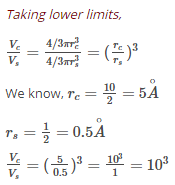Courses

# Test: Previous Year Questions - Surface Chemistry (Level 1)

## 8 Questions MCQ Test Chemistry Class 12 | Test: Previous Year Questions - Surface Chemistry (Level 1)

Description
This mock test of Test: Previous Year Questions - Surface Chemistry (Level 1) for Class 12 helps you for every Class 12 entrance exam. This contains 8 Multiple Choice Questions for Class 12 Test: Previous Year Questions - Surface Chemistry (Level 1) (mcq) to study with solutions a complete question bank. The solved questions answers in this Test: Previous Year Questions - Surface Chemistry (Level 1) quiz give you a good mix of easy questions and tough questions. Class 12 students definitely take this Test: Previous Year Questions - Surface Chemistry (Level 1) exercise for a better result in the exam. You can find other Test: Previous Year Questions - Surface Chemistry (Level 1) extra questions, long questions & short questions for Class 12 on EduRev as well by searching above.
QUESTION: 1

### Adding alum to turbid water, impurties are removed due to -  [AIEEE-2002]

Solution:

Turbid waters, containing colloidal particles, are normally treated by coagulation-flocculation followed by clarification. Generally, alum is the first coagulant of choice because of its lower cost and its widespread availability. The coagulation performance of Alum, an inorganic chemical coagulant was tested on synthetic high turbid water.

Results showed that coagulation process could remove turbidity effectively using relatively low levels of Alum.

QUESTION: 2

### Which one of the following characteristics is not correct for physical adsorption ?       [AIEEE-2003]

Solution:

The correct answer is Option D.
Physical adsorption increases with decrease in temperature as  easily liquefiable gases can adsorb more.
So option D is a false statement.

QUESTION: 3

### The volume of a colloidal particle, VC as compared to the volume of a solute particle in a true solution VS, could be –  [AIEEE-2005]

Solution:

For a true solution, the diameter range is 1 to < 10 Angstrom, and for colloidal solution,diameter range is 10-1000 Angstrom.QUESTION: 4

The disperse phase in colloidal iron (III) hydroxide and colloidal gold is positively and negatively charged respectively. Which of the following statements is not correct?

[AIEEE-2005]

Solution:

The dispersed phase in colloidal iron (III) hydroxide and colloidal gold is positively and negatively charged respectively.
When two solutions are mixed, they will affect mutual coagulation. This is because the charge on one sol is neutralized by the opposite charge on the other sol. Hence, statement A is not correct.

QUESTION: 5

In Langmuir's model of adsorption of a gas on a solid surface –

[AIEEE 2006]

Solution:

The correct answer is option B
According to Langmuir's adsorption isotherm, the mass of gas striking a given area of surface is proportional to the pressure of the gas as x/m​=k′p/​1+kp

QUESTION: 6

Gold numbers of protective colloids A, B, C and D are 0.50, 0.01, 0.10 and 0.005, respectively. The correct order of their protective powers is

[AIEEE 2008]

Solution:

The correct answer is option B
Gold numbers of protective colloids A,B,C and D are 0.50,0.01,0.10 and 0.005 respectively. The correct order of their protective powers is A(0.50)<C(0.10)<B(0.01)<D(0.005)
Higher is the value of gold number, lower is the protective power.

QUESTION: 7

Which of the following statements is incorrect regarding physissorptions ?

[AIEEE 2009]

Solution:

Physical adsorption is an exothermic process (i.e., ΔH = –ve) but its value is quite low because the attraction of gas molecules and solid surface is weak van der Waals forces.

QUESTION: 8

The coagulating power of electrolytes having ions Na+, Al3+ and Ba2+ for arsenic sulphide sol increases in the order:

[IIT Mains 2013]

Solution:

The correct is Option D.
According to Hardy Schulze rule, greater the charge on oppositely charged ions, greater is its coagulating power. Since arsenic sulphide is a negatively charged sol, thus, the order of coagulating power is :                       Na+ < Ba2+ < Al3+